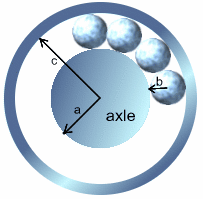#### You may also like### Overarch 2

Bricks are 20cm long and 10cm high. How high could an arch be built without mortar on a flat horizontal surface, to overhang by 1 metre? How big an overhang is it possible to make like this?### Cushion Ball

The shortest path between any two points on a snooker table is the straight line between them but what if the ball must bounce off one wall, or 2 walls, or 3 walls?### Stonehenge

Explain why, when moving heavy objects on rollers, the object moves twice as fast as the rollers. Try a similar experiment yourself.

# Ball Bearings

##### Age 16 to 18Challenge Level

The wheels of a car, or a bicycle, run smoothly because they are separated from the axle of the wheel by a ring of ball bearings as illustrated below. Of course, the wheel turns smoothly because the ball bearings fit exactly between the hub of the wheel and the axle with no room to move about except, of course, to rotate. It is this rotation that keeps the friction to a minimum, and so makes the wheel turn smoothly.Suppose that $a$ is the radius of the axle, $b$ is the radius of each ball-bearing, and $c$ is the radius of the hub (see the figure).What are the ratios ${a\over b}$, ${b\over c}$ and ${c\over a}$ when there are exactly three ball-bearings? What are these ratios when there are exactly four ball-bearings? Try to explain why the number of ball bearings determines the ratio ${c\over a}$ exactly. Can you find a formula for ${c\over a}$ in terms of $n$ when there are exactly $n$ ball-bearings?